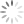Loading, please wait...# Universal functions Simple Arithmetics

a. Add: add() function is used to add the content of two or more arrays and return results in a new array.

#code to demonstrate the working of ufunc add().

``````import numpy as np

arry1 = np.array([12,13,14,15,16])

arry2 = np.array([22,23,24,25,26])

print(arrsum)

#output:[34 36 38 40 42]

``````

b. Subtract:  To perform subtraction on NumPy arrays, subtract() function can be used. This function subtracts the value of the second array from first and returns the result in a new array.

#code to demonstrate the working of ufunc subtract().

``````import numpy as np

#ufunc subtract

arry1 = np.array([12,13,14,15,16])

arry2 = np.array([22,23,24,25,26])

arrdiff = np.subtract(arry1,arry2)

print(arrdiff)

#output:[-10 -10 -10 -10 -10]``````

c. Multiply:

To perform multiplication on NumPy arrays, multiply() function can be used. This function multiplies the value of the second array from first and returns the result in a new array.

#code to demonstrate the working of ufunc multiply().

``````
import numpy as np

#ufunc multiply

arry1 = np.array([12,13,14,15,16])

arry2 = np.array([22,23,24,25,26])

arr = np.multiply(arry1,arry2)

print(arr)

#output:[264 299 336 375 416]

``````

d. Divide:

divide() function returns true division of the input array , element-wise. it takes 2- arrays as input and returns a ndarray as output.

#code to demonstrate the working of the ufunc divide().

``````
import numpy as np

#ufunc divide

arry1 = np.array([12,13,14,15,16])

arry2 = np.array([22,23,24,25,26])

arr = np.divide(arry1,arry2)

print(arr)

#output:[0.54545455 0.56521739 0.58333333 0.6 0.61538462]

``````

e. Power:

power() function is used to find power. It returns first array elements raised to powers from the second array, element-wise.

#code to demonstrate the working of ufunc power().

``````

import numpy as np

#ufunc power

arry1 = np.array([1,3,4,5,6])

arr = np.power(arry1, 10)

print(arr)

#output:[1 59049 1048576 9765625 60466176]``````

f. Remainder: To find the remainder or mod, the remainder() function can be used. It returns the element-wise remainder of the division. Computes the remainder complementary to the ‘floor_divide’ function. It is equivalent to the python modulus operator “y1%y2 and has the same sign as the divisor has.

#code to demonstrate the working of ufunc remainder().

``````import numpy as np

#ufunc power

arry1= np.array([10,20,70,80,90])

arry2 = np.array([1,3,4,5,6])

arr = np.remainder(arry1, arry2)

print(arr)

#output:[0 2 2 0 0]

``````

g. Quotient and Remainder:

divmod() function is used to get the quotient as well as the remainder. this function takes two arrays as input and returns a tuple containing two output array for quotient and the remainder.

#code to demonstrate working of ufunc divmod().

``````import numpy as np

#ufunc power

arry1= np.array([10,20,70,80,90])

arry2 = np.array([1,3,4,5,6])

arr = np.divmod(arry1, arry2)

print(arr)

#output:(array([10, 6, 17, 16, 15], dtype=int32), array([0, 2, 2, 0, 0], dtype=int32))``````

h. Absolute:

To find the absolute of given number absolute() is used. The absolute value of a number is the value without considering its sign. abs() or absolute() both function returns the same. If we pass a complex number to abs() or absolute() function, it will return the magnitude of that complex number.

#code to demonstrate working of ufunc absolute().

``````import numpy as np

#ufunc absolute

arry1= np.array([-10,20,-70.9 ,80.1 ,90])

arr = np.absolute(arry1)

print(arr)

#output:[10. 20. 70.9 80.1 90. ]``````